Highlighted

## How to convert column value labels to values?

Hi,

I'm new to the community (but have used JMP since version 1), so I don't know where to put this question.

Have imported a file from SPSS (.sav) with several columns with value labels. I want to use the value labels (which are actual names) instead of the values (which are numbers). Recode seems not to have this function. Could anybody help? Thanks a lot in advance. Jens

1 ACCEPTED SOLUTION

Accepted Solutions
Highlighted

## Re: How to convert column value labels to values?

I could not resist try to script Karen's idea. Get value Labels return an expression with one argument: a list of "assignments", e.g.  2 = "No". I come to think of two approaches: either use these (illegal) assignments directly or as Xan suggests manipulate them into a Match expression.

Below are my attempts at both approaches. The Match approach is faster here. For large tables and if many values lack labels the performance appears to become more equal. The Match code can probably be be improved.

``````open_example_table = Expr(
dt = Open( "\$SAMPLE_DATA/CrabSatellites.jmp" );
col = Column( dt, "color" );
col << data type( character );
);

//1
open_example_table;
bt = HP Time();
// Direct assignment code
v = Arg( col << get value labels(), 1 );
For( i = 1, i <= N Items( v ), i++,
col[dt << get rows where( col[] == Arg( v[i], 1 ) )] = Arg( v[i], 2 )
);
// End direct assignment
ht = HP Time();
t1 = ht - bt;
Close( dt, no save );

// 2
open_example_table;
bt = HP Time();
// code for building Match expression by string manipulation
match_expr = Parse(
"Match( col[]," || Substitute( Char( Arg( col << get value labels(), 1 ) ),
"{", "",
"=", ",",
"}", ""
) || ",col[])"
);
For Each Row( col[] = match_expr );
// End Match
ht = HP Time();
t2 = ht - bt;

Show( t1, t2 );

t1 = 1729;
t2 = 1019;``````

//:*/

10 REPLIES 10
Highlightedstan_koprowskiCommunity Manager

## Re: How to convert column value labels to values?

Hello,

If I am understanding your question correctly.

You can turn the value labels on or off through the column properties.

In this example using the Consumer Preferences from the sample data I was able to right-click on the column and uncheck the value label option.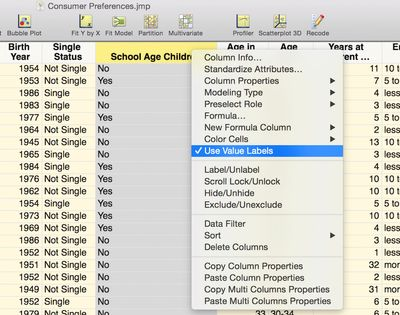The column is no longer using the value label as shown below.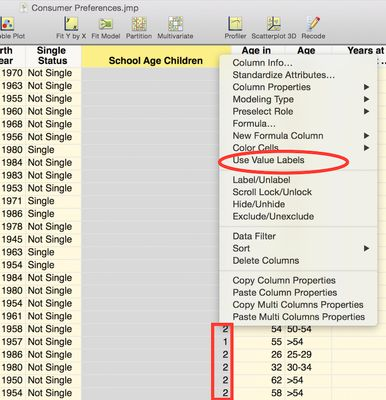I hope this helps.

Best,

Stan

Highlighted

## Re: How to convert column value labels to values?

Hi Stanley, Thanks for trying to help.  I know the function of value labels, but what I need is to change or substitute 1,2,3 values to No, Yes (e.g. as in your example) character values.

I need to join two tables with matching columns one of which has character values (same as the value labels in the other table, i.e., no coding was used), the other has numeric values (codes) with value labels.

Or is there a way to join the tables on the fly based on a column's value labels in one table and actual values in the matching column in the other table?

Best regards

Jens

Highlighted

## Re: How to convert column value labels to values?

Change Data Type to character and then use Recode.

Highlighted

## Re: How to convert column value labels to values?

Hi, thanks for the tip. There more than 300 value labels, which I would have to enter manually in Recode - I hoped there would be a smarter way.

Highlighted

## Re: How to convert column value labels to values?

If you have a table of the codes and their values you could use the UPDATE command to add the appropriate value column.

Highlighted

## Re: How to convert column value labels to values?

I think what we would like (but don't have right now) is a "recode with value labels".  I too have wanted this feature from time to time.

Karen

Highlighted

## Re: How to convert column value labels to values?

Good idea, Karen.

Jens, It's not easy to get the labels out as data. Two ways I can think of:

1. With scripting, you can use Get Value Labels and manually or programmatically manipulate the result to be like the Match() expression that Recode generates when you save it as a script.
2. Do a Tabulate on the variable and use Make Into Data Table on the result.

For #2, you'll need some way to match things up if they're not in a sorted one-to-one mapping. Steps for that:

1. Create a copy of the values in a new column (without Value Labels)
2.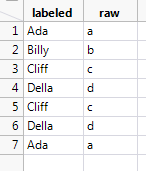3. Use Tabulate with both variables as category columns:
4.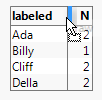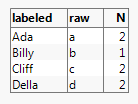5. Use the Tabulate command Make into Data Table, to get a new table with no Value Labels properties.
6. Use Tables > Join to match on the raw values and get the new "labeled" column without Value Labels.
Highlighted

## Re: How to convert column value labels to values?

I could not resist try to script Karen's idea. Get value Labels return an expression with one argument: a list of "assignments", e.g.  2 = "No". I come to think of two approaches: either use these (illegal) assignments directly or as Xan suggests manipulate them into a Match expression.

Below are my attempts at both approaches. The Match approach is faster here. For large tables and if many values lack labels the performance appears to become more equal. The Match code can probably be be improved.

``````open_example_table = Expr(
dt = Open( "\$SAMPLE_DATA/CrabSatellites.jmp" );
col = Column( dt, "color" );
col << data type( character );
);

//1
open_example_table;
bt = HP Time();
// Direct assignment code
v = Arg( col << get value labels(), 1 );
For( i = 1, i <= N Items( v ), i++,
col[dt << get rows where( col[] == Arg( v[i], 1 ) )] = Arg( v[i], 2 )
);
// End direct assignment
ht = HP Time();
t1 = ht - bt;
Close( dt, no save );

// 2
open_example_table;
bt = HP Time();
// code for building Match expression by string manipulation
match_expr = Parse(
"Match( col[]," || Substitute( Char( Arg( col << get value labels(), 1 ) ),
"{", "",
"=", ",",
"}", ""
) || ",col[])"
);
For Each Row( col[] = match_expr );
// End Match
ht = HP Time();
t2 = ht - bt;

Show( t1, t2 );

t1 = 1729;
t2 = 1019;``````

//:*/

Highlighted

## Re: How to convert column value labels to values?

Another way to convert the column to a column of labels, is to copy the column, paste the data into a script editor window (or any text window). Then copy the data from the text window. Paste into a new character column in your original table.

Article Labels

There are no labels assigned to this post.